# Electric Current

Charge, PD, Current and Resistance. IV Graphs.

Charge

·      Charge is a fundamental property of particles. It exists in two types that we label positive (+) and negative (-). Like charges repel, unlike charges attract

·      Charge carriers can be any charged particles e.g. ions in solution, molten metal but more usually electrons in conductors.=

·      The unit of charge is the Coulomb (C).

1 electron has a charge of 1.60 x 10-19C

Therefore 1/1.60 x 10-19C = 6.25 x 1018 electrons have 1 Coulomb of charge

Current

·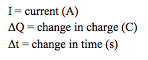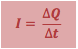A current is a rate of flow of charge i.e. how many Coulombs of charge pass a point per second measured in Amperes or ‘Amps’, A·      Current is measured by an ammeter in series

·      An ideal ammeter has zero resistance

Potential Difference

·P.d. is the amount of energy per Coulomb of charge

·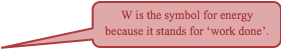The unit of p.d. is therefore JC-1 which we call the Volt, V

·P.d. is measured by a voltmeter in parallel ‘across’ a component in a circuit as the energy transferred per coulomb of charge. All electrical components transfer some energy in order to operate e.g. a bulb, a speaker, a motor.

·      An ideal voltmeter has infinite resistance (so no current flows through it)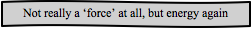E.M.F (Electro-motive force)

·      A battery or power pack transfers energy from another source into electrical energy. The amount of energy (or work) it passes on to each coulomb of charge is called the E.m.f., _ also measured in JC-1 or Volts, V

Power

·When there is a p.d. across a component and a current is flowing through it, some energy is transferred. The rate at which this happens is called the power in Js-1 or Watts (W)

·      To calculate the total energy transferred to a device in a given time, E = Power(W) x time(s)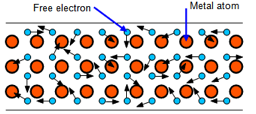Resistance

·      Free electrons move randomly with thermal energy until a p.d. is applied

·      When a current flows they move in the direction of the positive terminal of the source

·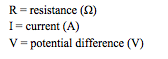They are impeded by the metal ions in the wire causing their path to deviate, and some energy to be transferred to the ions in the lattice structure of the metal

·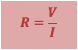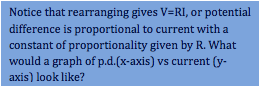This is resistance and the unit of measurement is the Ohm (Ω)

·An ohmic conductor is one where the p.d. is proportional to the current at constant temperature

·      The longer a wire the greater its resistance

·      The larger the cross sectional area of a wire the less its resistance

·      In a metal, resistance increases with temperature

Resistivity

·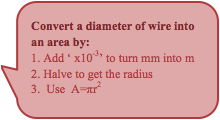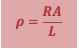To compare the electrical properties of different materials without the dimensions of the sample having an effect, the quantity resistivity measured in units of Ωm  is defined as:

Superconductor

·      A superconductor is a material with zero resistance (and also resistivity) below a certain critical temperature

IV Characteristics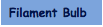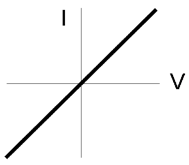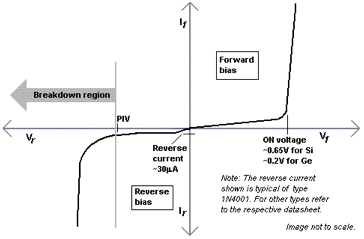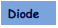To do:

·      Explain and learn each of the IV graphs above

·      Draw and learn circuit symbols for the following: thermistor, Light Dependent Resistor, Light Emitting Diode.

·      How does the resistance of a thermistor change with temperature?

·      How does the resistance of an L.D.R. change with light intensity?